# Consider a two-dimensional ?ow with velocity components   .Find expressions for the vorticity and the strain rate...

Consider a two-dimensional ?ow with velocity
components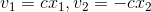.Find expressions for the
vorticity and the strain rate tensor.

V1 = CT1, 12 = -CT2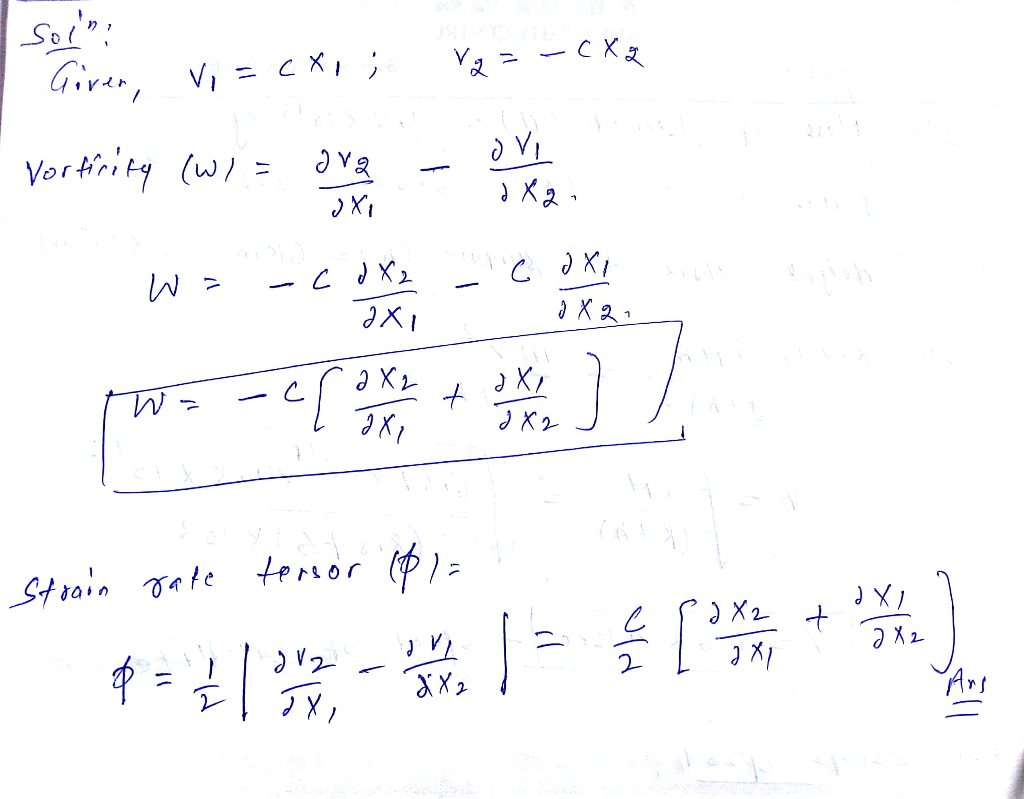#### Earn Coin

Coins can be redeemed for fabulous gifts.

Similar Homework Help Questions
• ### Question 1 (10 points) Rotational Flow and Vorticity The velocity components for a two-dimensional flow are...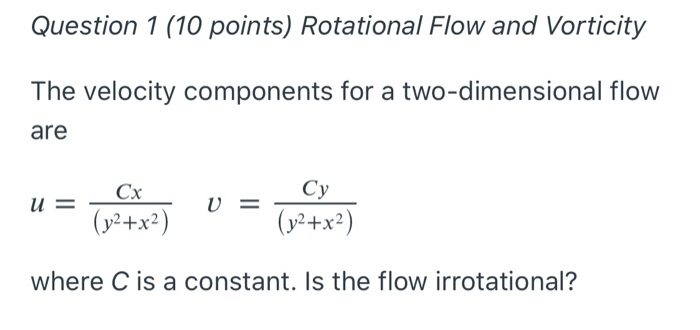Question 1 (10 points) Rotational Flow and Vorticity The velocity components for a two-dimensional flow are u = ln hie) v = created where C is a constant. Is the flow irrotational?

• ### Consider a steady, two-dimensional, incompressible flow field in the x-y plane. The linear strain rate in...

Consider a steady, two-dimensional, incompressible flow field in the x-y plane. The linear strain rate in the x-direction is 1.8 s−1. Calculate the linear strain rate in the y-direction. The linear strain rate in the y-direction is

• ### Consider a steady, two-dimensional, incompressible flow field in the x-y plane. The linear strain rate in...

Consider a steady, two-dimensional, incompressible flow field in the x-y plane. The linear strain rate in the x-direction is 1.65 s−1. Calculate the linear strain rate in the y-direction. The linear strain rate in the y-direction is   s−1

• ### Consider a velocity field given by: Axi 2 a) Find the strain rate and rotation rate...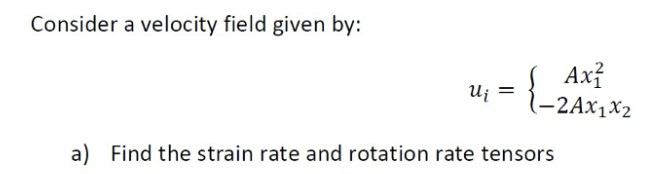Consider a velocity field given by: Axi 2 a) Find the strain rate and rotation rate tensors

• ### 3. (30 points) Consider a two-dimensional flow of a Newtonian fluid in which the velocity field...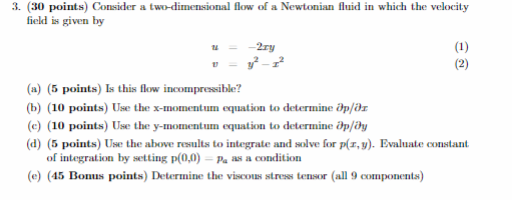3. (30 points) Consider a two-dimensional flow of a Newtonian fluid in which the velocity field is given by 2ry (a) (5 points) Is this flow incompressible? b) (10 points) Use the x-momentum equation to determine ap/aa (e) (10 points) Use the y-momentum equation to determine ap/dy (d) (5 points) Use the above results to integrate and solve for p(x, y). Evaluate constant of integration by setting p(0,0- Pa as a condition (e) (45 Bonus points) Determine the viscouas stress...

• ### 1. A general equation for a steady, two-dimensional velocity field that is linear in both spatial...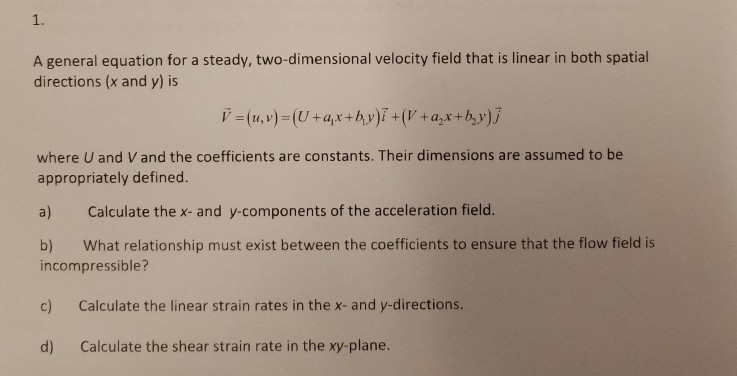1. A general equation for a steady, two-dimensional velocity field that is linear in both spatial directions (x and y) is h) where U and V and the coefficients are constants. Their dimensions are assumed to be appropriately defined. a) Calculate the x- and y-components of the acceleration field b) What relationship must exist between the coefficients to ensure that the flow field is incompressible? c) Calculate the linear strain rates in the x- and y-directions. d) Calculate the shear...

• ### Problem #5 Consider a steady, incompressible, inviscid two-dimensional flow in a corner, the stream function is...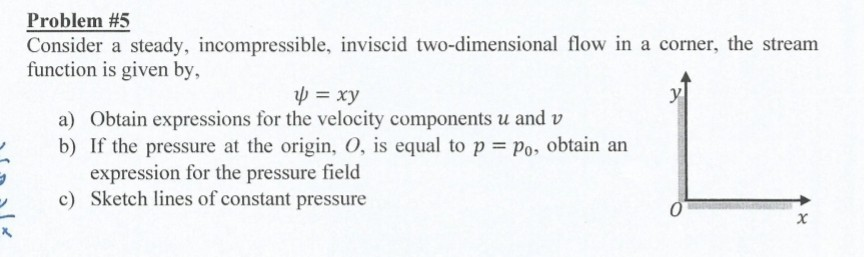Problem #5 Consider a steady, incompressible, inviscid two-dimensional flow in a corner, the stream function is given by, -xy a) Obtain expressions for the velocity components u and v b) If the pressure at the origin, O, is equal to p o obtain an expression for the pressure field Sketch lines of constant pressure c)

• ### (30p) Consider a two-dimensional strain state defined by 612 = € and all normal strains zero....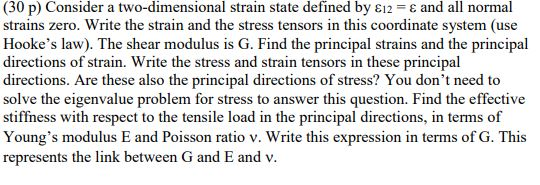(30p) Consider a two-dimensional strain state defined by 612 = € and all normal strains zero. Write the strain and the stress tensors in this coordinate system (use Hooke's law). The shear modulus is G. Find the principal strains and the principal directions of strain. Write the stress and strain tensors in these principal directions. Are these also the principal directions of stress? You don't need to solve the eigenvalue problem for stress to answer this question. Find the effective...

• ### A general equation for a steady, two-dimensional velocity field that is linear in both spatial directions...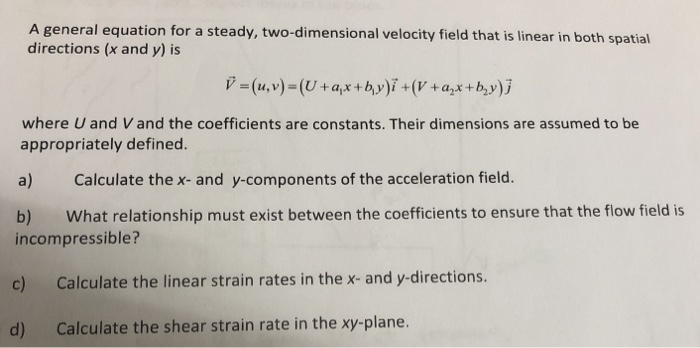A general equation for a steady, two-dimensional velocity field that is linear in both spatial directions (x and y) is -(u,v)-(U+a+by)+(Va+b,y)j where U and V and the coefficients are constants. Their dimensions are assumed to be appropriately defined. a) Calculate the x- and y-components of the acceleration field. b) What relationship must exist between the coefficients to ensure that the flow field is incompressible? c) Calculate the linear strain rates in the x- and y directions. d) Calculate the shear...

• ### 1. The velocity in a certain two-dimensional flow field is given by the equation -= 2zt의...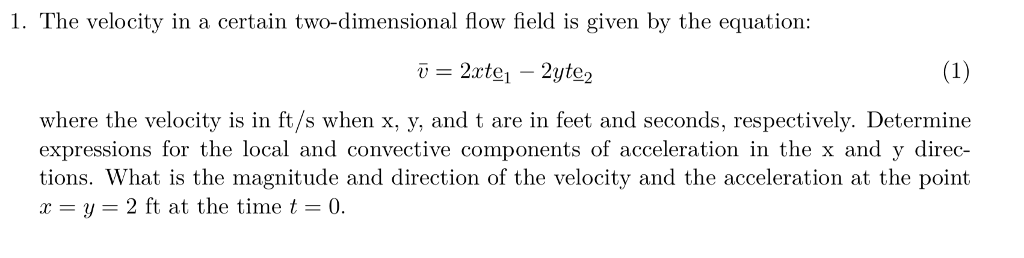1. The velocity in a certain two-dimensional flow field is given by the equation -= 2zt의 _ 2yte2 where the velocity is in ft/s when x, y, and t are in feet and seconds, respectively. Determine expressions for the local and convective components of acceleration in the x and y direc tions. What is the magnitude and direction of the velocity and the acceleration at the point x = y = 2 ft at the time t = 0.